## 2.19Energy and temperature

Speciﬁc internal energy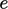and temperature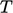were described in Sec. 2.17 and Sec. 2.16 , respectively. They are related through the speciﬁc heat capacity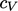, deﬁned by Eq. (2.61 ) in Sec. 2.18 .

Analyses involving heat usually incorporate both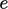and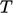since:

•is the measurable quantity speciﬁed as initial and boundary data and whose data is required as part of the “results”;
•is the calculated quantity solved in energy conservation, e.g. Eq. (2.51 ), but whose data is usually of no interest.

Conversion of values betweenandis therefore needed, and vice versa. Incorporating Eq. (2.61 ) into a deﬁnite integral for,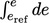, gives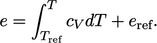(2.62)
The terms in Eq. (2.62 ) are illustrated below on a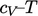graph. Energy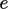is represented by the area under the curve, in which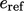represents a reference energy up to a reference temperature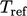, and the integral fromto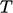is shown by the shaded area.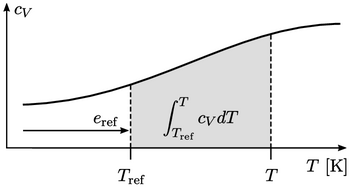For applications that cover a reasonably narrow temperature range,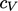can be assumed constant. From Eq. (2.62 ), the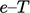relation becomes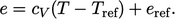(2.63)
Alternatively,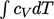can be integrated analytically by representing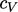by a polynomial of order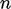with coeﬃcients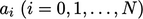ﬁtted to measured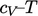data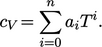(2.64)
The values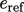and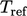ultimately add a constant component to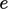. Since Eq. (2.51 ) is concerned with changes inand the absolute valuesare usually of no interest, the values ofandare often immaterial.

The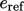and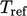values become important when the composition of a ﬂuid changes due to the mixing of constituent ﬂuid species, e.g.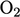,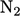, or chemical reactions, e.g. with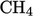. Each ﬂuid specie possesses a diﬀerent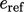so any change to the specie concentrations will change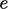of the overall ﬂuid.

In those circumstances,is commonly represented by the heat of formation per unit mass,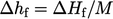. The standard heat of formation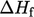is the change of enthalpy during the formation of 1 mole of a substance from its constituent elements at standard temperature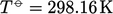. Measured heats of formation are available for numerous ﬂuid species.20

If an analysis involves changes to ﬂuid composition, it can then adopt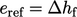and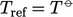for individual ﬂuid species, to account for the change in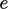due to changes in the concentrations of ﬂuid species.

20Alexander Burcat and Branko Ruscic, Third Millennium ideal gas and condensed phase thermochemical database for combustion, 2005.

Notes on CFD: General Principles - 2.19 Energy and temperature